# Invited Speakers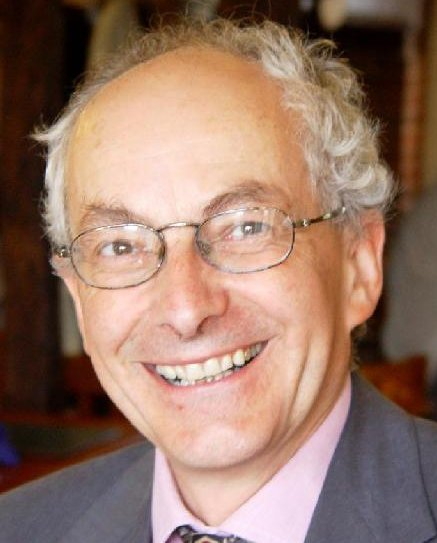Martin Hyland Achim Jung Paul-André Melliès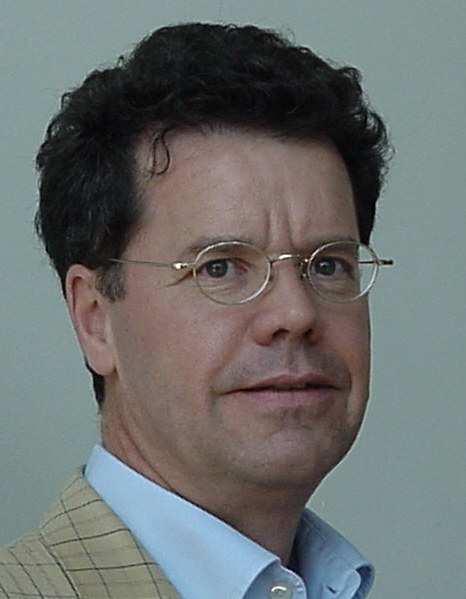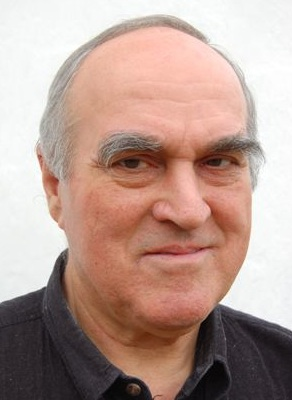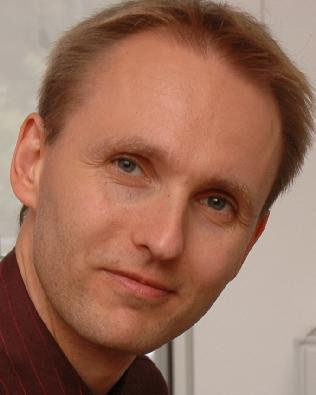Ieke Moerdijk Aleš Pultr Lutz Schroeder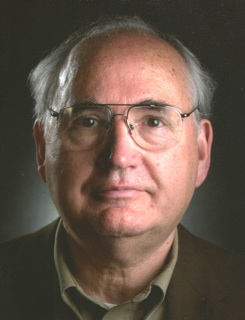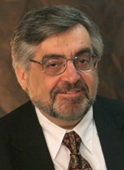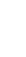Dana Scott Kazushige Terui Constantine Tsinakis

## Invited Talks and Abstracts

(Click on the title of the talk to download the presentation/paper of the talk)

Martin Hyland, Multicategories in and around algebra and logic. An algebraic theory is a one object cartesian multicategory. An interpretation of the lambda calculus (with beta-eta equality) is a one-object cartesian closed multicategory. This talk will be in the first place a plea for an appreciation of the role of multicategories. Secondly I shall develop a general perspective within which one can explain many variations on the multicategory notion. The constructions are interesting in themselves but also provide models of Linear Logic. If I get time I shall say something about the differential calculus from this point of view.

Achim Jung, On domain algebras.

Paul-Andre Mellies, Logical proofs as topological knots. Both logical proofs and topological knots are symbolic structures considered up to a notion of transformation: cut-elimination in the case of proofs, topological deformation in the case of knots. In this lecture, I will explain how to unify the two topics in the language of category theory. More precisely, I will advocate that game semantics offers the proof theoretic counterpart of what is sometimes called functorial knot theory. This point of view leads to a purely topological formulation of proofs, clarifying the nature of logical duality, and the basic concepts of game semantics: dialogue games, innocence, views, plays.

Ieke Moerdijk, Topological constructions and derived rules. In this talk, I will sketch some topological proofs of proof-theoretic properties of intuitionistic set theory such as "every provable function from the reals to itself is provably continuous", "every provable open cover of Cantor space has a provable finite subcover", and rules like these. Within the spirit of the conference, I'll explain how these topological proofs involve the algebraisation of topology (via locale theory) and the construction of models for set theory using categories of sheaves. I will also discuss to what extent these proofs apply to predicative systems of set theory (ongoing work with Benno van den Berg.)

Ales Pultr, Some logical aspects of Priestley duality. (Talk based on joint work with R.N. Ball and J. Sichler) Recall the Priestley duality, a contravariant equivalence of the category of Priestley spaces (that is, ordered compact Hausdorff spaces, order-totally-disconnected) and the category of bounded distributive lattices. It was observed that prohibiting some configurations (induced finite connected posets) in the spaces gave rise to first-order theories of the corresponding distributive lattices. Later, it turned out to be true for all acyclic configurations; on the other hand it has been shown (almost) that it is never the case for the cyclic ones. The talk will be concerned with this phenomenon and some related questions. First we will discuss the case of the topped configurations where one has a complete proof that the acyclicity is a necessary and sufficient condition for the first order definability. Then we will speak about the general case. Here one has a positive result for acyclic configurations again, but the cyclicity still leaves some open problems. We will speak about what is known, and point out the difficulties obstructing a complete solution. In the conclusion we will briefly mention some theories that arise in special cyclic cases.

Lutz Schroeder, Coalgebraic Logics. The field of modal logic, in particular where it intersects with symbolic methods in artificial intelligence, has evolved semantically to encompass wider classes of structures beyond classical Kripke-style relational structures. Modal logics such as graded modal logic, coalition logic, probabilistic modal logic, or conditional logic are interpreted over semantic structures ranging from Markov chains over game frames to selection function models. Coalgebraic modal logic has emerged as a unifying framework that allows for a uniform metatheory of such logics, with generic results including expressiveness, completeness, small model properties, decidability, and complexity. We give an overview of these results and illustrate their basic flavour; we conclude with an outlook on recent results on coalgebraic logics with nominals.

Dana S. Scott, Mixing Modality and Probability. Orlov first  and Gödel later  pointed out the connection between the Lewis System S4 and Intuitionistic Logic. McKinsey and Tarski gave an algebraic formulation and proved completeness theorems for propositional systems using as models topological spaces with the interior operator corresponding to the necessitation modality. Earlier, Tarski and Stone had each shown that the lattice of open subsets of a topological space models intuitionistic propositional logic. Expanding on a suggestion of Mostowski about interpreting quantifiers, Rasiowa and Sikorski used the topological models to model first-order logic. After the advent of Solovey''s recasting of Cohen''s independence proofs as using Boolean-valued models, topological models for modal higher- order logic were studied by Gallin and others. (This very, very brief history does not attempt to acknowledge legions of other researchers and investigations of logics other than S4.) For Boolean-valued logic, the complete Boolean algebra Meas([0,1])/Null of measurable subsets of the unit interval modulo sets of measure zero gives every proposition a probability. Perhaps not as well known is the observation that the measure algebra also carries a nontrivial S4 modality defined with the aid of the sublattice Open([0,1])/Null of open sets modulo null sets. This sublattice is closed under arbitrary joins and finite meets in the measure algebra, but it is not the whole of the measure algebra. Consideration of this model of modality brings up several questions: (1) What completeness results can be expected in the first- order case? (2) How does this model differ from models used by Montague and Gallin for higher-order logic? (3) In employing this model to interpret notions of extensional and intensional functions, what revision of the definition of a topos is appropriate? (4) What kind of definition of random real number should be chosen to go along with the inherent probability? (5) Will the measure-preserving automorphisms of the modal measure algebra give us a connection between properties of the logic and the results of Ergodic Theory?

Kazushige Terui, Algebraic proof theory for substructural logics II. I will outline an algebraic and systematic approach to proof theory for substructural logics, which is being developed together with A. Ciabattoni, N. Galatos and L. Strassburger. In proof theory, one transforms Hilbert axioms into Gentzen rules to obtain cut-elimination theorems. In algebra, one embeds a given algebra into a complete one for various purposes. It is not surprising that these two are deeply related, since both are concerned with conservative extension. The connection is, however, even tighter than expected. For one thing, the passage from Hilbert axioms to Gentzen rules plays a crucial role for algebraic completion too. For another, Maehara-Okada cut-elimination can be construed as generalization of Dedekind-MacNeille completion. To illustrate the situation, we introduce a hierarchy on nonclassical axioms. One of our goals is then to identify a boundary in the hierarchy, below which proof theory (cut-elimination) works well and above which one finds negative algebraic results (failure of completions). Besides the general issue, I will also discuss a curious borderline case, that is abelian logic of Meyer and Slaney. It is a logic for compact closed categories with binary products and coproducts. Even though the corresponding variety of lattice-ordered abelian groups is not closed under completions, it has a cut-free hypersequent calculus discovered by Metcalfe, Olivietti and Gabbay. With this example, I will illustrate how our theory works as a heuristic principle for finding a cut-free (hyper)sequent calculus, and also how it might be useful in a categorical setting as well.

Costas Tsinakis, Interpolation and amalgamation in ordered algebras. The connection between interpolation theorems in logic and variants of the amalgamation property in algebra is well known. This talk, based on joint work with George Metcalfe and Franco Montagna, provides a fresh perspective and new techniques in the study of these properties in a universal algebraic setting. Further, it explores the application of these techniques to varieties of residuated lattices and their logical counterparts, the so called substructural logics.